modelAccuracyPlot

Scatter plot of predicted and observed EADs

Description

example

modelAccuracyPlot(eadModel,data) returns a scatter plot of observed vs. predicted exposure at default (EAD) data with a linear fit. modelAccuracyPlot supports comparison against a reference model. By default, modelAccuracyPlot plots in the EAD scale.

example

modelAccuracyPlot(___,Name,Value) specifies options using one or more name-value pair arguments in addition to the input arguments in the previous syntax. You can use the ModelLevel name-value pair argument to compute metrics using the underlying model's transformed scale.

example

h = modelAccuracyPlot(ax,___,Name,Value) specifies options using one or more name-value pair arguments in addition to the input arguments in the previous syntax and returns the figure handle h.

Examples

collapse all

This example shows how to use fitEADModel to create a Tobit model and then use modelAccuracyPlot to generate a scatter plot for predicted and observed EADs.

ans=8×6 table
UtilizationRate    Age     Marriage        Limit         Drawn          EAD
_______________    ___    ___________    __________    __________    __________

0.24359        25     not married         44776         10907         44740
0.96946        44     not married    2.1405e+05    2.0751e+05         40678
0        40     married        1.6581e+05             0    1.6567e+05
0.53242        38     not married    1.7375e+05         92506        1593.5
0.2583        30     not married         26258        6782.5        54.175
0.17039        54     married        1.7357e+05         29575        576.69
0.18586        27     not married         19590          3641        998.49
0.85372        42     not married    2.0712e+05    1.7682e+05    1.6454e+05

rng('default');
c = cvpartition(NumObs,'HoldOut',0.4);
TrainingInd = training(c);
TestInd = test(c);

Select Model Type

Select a model type for Tobit or Regression.

ModelType =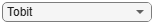"Tobit";

Select Conversion Measure

Select a conversion measure for the EAD response values.

ConversionMeasure =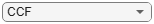"CCF";

Tobit with properties:

CensoringSide: "right"
LeftLimit: NaN
RightLimit: 1
ModelID: "Tobit"
Description: ""
UnderlyingModel: [1x1 risk.internal.credit.TobitModel]
PredictorVars: ["UtilizationRate"    "Age"    "Marriage"]
LimitVar: "Limit"
DrawnVar: "Drawn"
ConversionMeasure: "ccf"

Display the underlying model. The underlying model's response variable is the transformation of the EAD response data. Use the 'LimitVar' and 'DrawnVar' name-value arguments to modify the transformation.

Tobit regression model, right-censored:
Y* ~ 1 + UtilizationRate + Age + Marriage

Estimated coefficients:
Estimate        SE         tStat        pValue
__________    _________    ________    __________

(Intercept)                0.48416      0.11449      4.2289    2.3966e-05
UtilizationRate            -1.6791     0.074337     -22.588             0
Age                     -0.0035653    0.0026785     -1.3311       0.18323
Marriage_not married     -0.020553     0.048875    -0.42053       0.67412
(Sigma)                     1.5317     0.016933      90.455             0

Number of observations: 4378
Number of left-censored observations: 0
Number of uncensored observations: 4377
Number of right-censored observations: 1
Log-likelihood: -10471.6

EAD prediction operates on the underlying compact statistical model and then transforms the predicted values back to the EAD scale. You can specify the predict function with different options for the 'ModelLevel' name-value argument.

For model validation, use modelDiscrimination, modelDiscriminationPlot, modelAccuracy, and modelAccuracyPlot.

Use modelDiscrimination and then modelDiscriminationPlot to plot the ROC curve.

ModelLevel =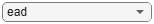"ead";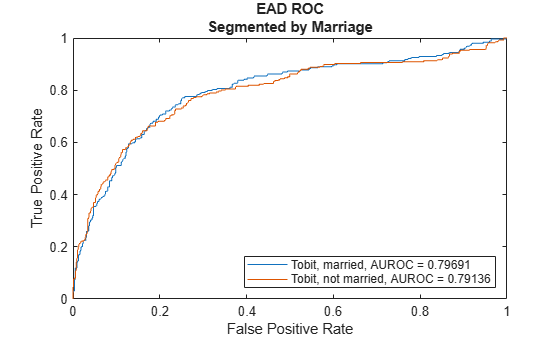Use modelAccuracy and then modelAccuracyPlot to show a scatter plot of the predictions.

YData =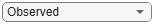"Observed";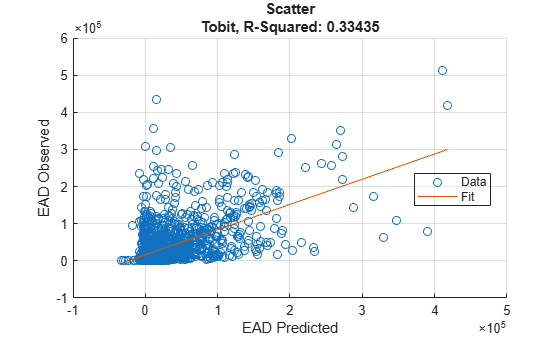Input Arguments

collapse all

Exposure at default model, specified as a previously created Regression or Tobit object using fitEADModel.

Data Types: object

Data, specified as a NumRows-by-NumCols table with predictor and response values. The variable names and data types must be consistent with the underlying model.

Data Types: table

(Optional) Valid axis object, specified as an ax object that is created using axes. The plot will be created in the axes specified by the optional ax argument instead of in the current axes (gca). The optional argument ax must precede any of the input argument combinations.

Data Types: object

Name-Value Arguments

Specify optional comma-separated pairs of Name,Value arguments. Name is the argument name and Value is the corresponding value. Name must appear inside quotes. You can specify several name and value pair arguments in any order as Name1,Value1,...,NameN,ValueN.

Data set identifier, specified as the comma-separated pair consisting of 'DataID' and a character vector or string. The DataID is included in the output for reporting purposes.

Data Types: char | string

Model level, specified as the comma-separated pair consisting of 'ModelLevel' and a character vector or string.

Note

Regression models support all three model levels, but a Tobit model supports model levels only for 'ead' and 'conversionMeasure'.

Data Types: char | string

EAD values predicted for data by the reference model, specified as the comma-separated pair consisting of 'ReferenceEAD' and a NumRows-by-1 numeric vector. The scatter plot output is plotted for both the eadModel object and the reference model.

Data Types: double

Identifier for the reference model, specified as the comma-separated pair consisting of 'ReferenceID' and a character vector or string. 'ReferenceID' is used in the scatter plot output for reporting purposes.

Data Types: char | string

Data to plot on x-axis, specified as the comma-separated pair consisting of 'XData' and a character vector or string for one of the following:

• 'predicted' — Plot the predicted EAD values in the x-axis.

• 'observed' — Plot the observed EAD values in the x-axis.

• 'residuals' — Plot the residuals in the x-axis.

• VariableName — Use the name of the variable in the data input, not necessarily a model variable, to plot in the x-axis.

Data Types: char | string

Data to plot on y-axis, specified as the comma-separated pair consisting of 'YData' and a character vector or string for one of the following:

• 'predicted' — Plot the predicted EAD values in the y-axis.

• 'observed' — Plot the observed EAD values in the y-axis.

• 'residuals' — Plot the residuals in the y-axis.

Data Types: char | string

Output Arguments

collapse all

Figure handle for the scatter and line objects, returned as handle object.

collapse all

Model Accuracy Plot

The modelAccuracyPlot function returns a scatter plot of observed vs. predicted loss given default (EAD) data with a linear fit and reports the R-square of the linear fit.

The XData name-value pair argument allows you to change the x values on the plot. By default, predicted EAD values are plotted in the x-axis, but predicted EAD values, residuals, or any variable in the data input, not necessarily a model variable, can be used as x values. If the selected XData is a categorical variable, a swarm chart is used. For more information, see swarmchart.

The YData name-value pair argument allows users to change the y values on the plot. By default, observed EAD values are plotted in the y-axis, but predicted EAD values or residuals can also be used as y values. YData does not support table variables.

The linear fit and reported R-squared value always correspond to the linear regression model with the plotted y values as response and the plotted x values as the only predictor.

 Baesens, Bart, Daniel Roesch, and Harald Scheule. Credit Risk Analytics: Measurement Techniques, Applications, and Examples in SAS. Wiley, 2016.

 Bellini, Tiziano. IFRS 9 and CECL Credit Risk Modelling and Validation: A Practical Guide with Examples Worked in R and SAS. San Diego, CA: Elsevier, 2019.

 Brown, Iain. Developing Credit Risk Models Using SAS Enterprise Miner and SAS/STAT: Theory and Applications. SAS Institute, 2014.

 Roesch, Daniel and Harald Scheule. Deep Credit Risk. Independently published, 2020.